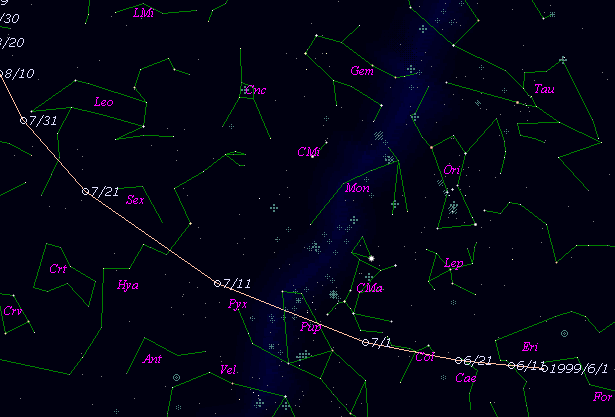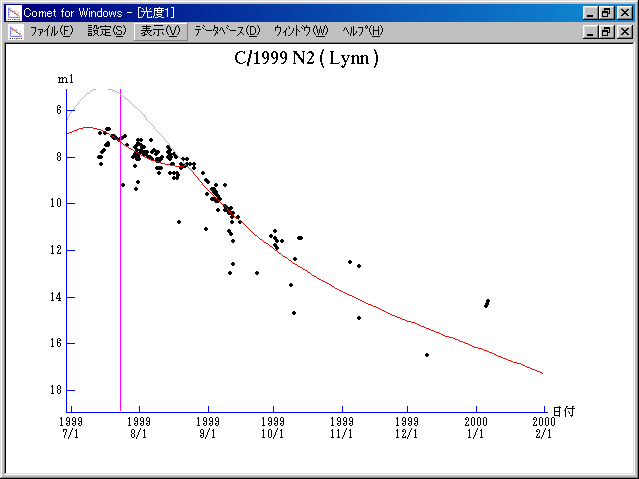\$B%j%sWB@1(B

C/1999 N2 ( Lynn )\$B%W%m%U%#!<%k(B

 \$BH/8+F|(B 1999\$BG/(B 7\$B7n(B13\$BF|(B \$BH/8+8wEY(B 8.0\$BEy(B \$BH/8+ D. W. Lynn (near Melbourne)\$B\$B50F;MWAG(B

```   The following improved orbital elements by Kenji Muraoka, are
from 176 observations  1999 July 14 to Sept. 11, perturbations
by 9 Planets, Moon and 5 minor planets were taken into account.
The mean residual is +/- 0.73 arc seconds.

Epoch  =  1999 Aug. 10.0  TT       JDT = 2451400.5
T  =  1999 July 23.05050       +/- 0.00011 (m.e.) TT
Peri. =  357.83403                +/- 0.00019
Node  =  254.67569                +/- 0.00021   (2000.0)
Incl. =  111.65609                +/- 0.00018
q  =    0.7612843              +/- 0.0000002 AU
e  =    0.9974591              +/- 0.0000202
1/a  =   +0.0033376              +/- 0.0000265 1/AU
( P  =   5186 years )
orig. 1/a  =   +0.003443
fut.  1/a  =   +0.004352
```\$B@1?^(B\$B8wEYJQ2=(B

```        m1 = 7.5 + 5 log\$B&\$(B -  5   log r  [  ,30]  (              \$B!A(B1999\$BG/(B 8\$B7n(B22\$BF|(B)
m1 = 7.8 + 5 log\$B&\$(B + 15.0 log r  [30,  ]  (1999\$BG/(B 8\$B7n(B22\$BF|!A(B              )

\$B"((B \$B3%?'\$N6J@~\$O!"(Bm1 = 7.8 + 5 log\$B&\$(B + 15.0 log r \$B\$N8wEY6J@~\$G\$9!#(B
```\$B50F;MWAG\$OB<2,7r<#;a\$N7W;;\$K\$h\$k\$b\$N\$G\$9!#(B \$B@1?^\$O(B StellaNavigator Ver.2.0 for Windows (\$B%"%9%H%m%"!<%D(B \$BJTCx(B / \$B%"%9%-!<=PHG6I4)(B) \$B\$G:n@.\$7\$?\$b\$N\$G\$9!#(B \$B8wEY%0%i%U\$O(BComet for Windows\$B\$G:n@.\$7\$?\$b\$N\$G\$9!#(B